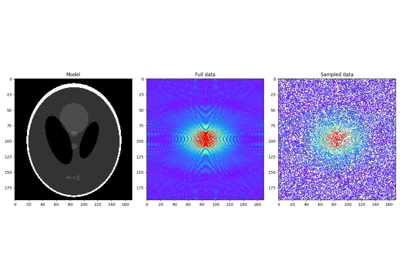# pyproximal.optimization.pnp.PlugAndPlay#

pyproximal.optimization.pnp.PlugAndPlay(proxf, denoiser, dims, x0, solver=<function ADMM>, **kwargs_solver)[source]#

Plug-and-Play Priors with any proximal algorithm of choice

Solves the following minimization problem using any proximal a lgorithm of choice:

$\mathbf{x},\mathbf{z} = \argmin_{\mathbf{x}} f(\mathbf{x}) + \lambda g(\mathbf{x})$

where $$f(\mathbf{x})$$ is a function that has a known gradient or proximal operator and $$g(\mathbf{x})$$ is a function acting as implicit prior. Implicit means that no explicit function should be defined: instead, a denoising algorithm of choice is used. See Notes for details.

Parameters
proxfpyproximal.ProxOperator

Proximal operator of f function

denoiserfunc

Denoiser (must be a function with two inputs, the first is the signal to be denoised, the second is the tau constant of the y-update in PlugAndPlay)

dimstuple

Dimensions used to reshape the vector x in the prox method prior to calling the denoiser

x0numpy.ndarray

Initial vector

solverpyproximal.optimization.primal or pyproximal.optimization.primaldual

Solver of choice

kwargs_solverdict

Additonal parameters required by the selected solver

Returns
out

Output of the solver of choice

Notes

Plug-and-Play Priors  can be used with any proximal algorithm of choice. For example, when ADMM is selected, the resulting scheme can be expressed by the following recursion:

$\begin{split}\mathbf{x}^{k+1} = \prox_{\tau f}(\mathbf{z}^{k} - \mathbf{u}^{k})\\ \mathbf{z}^{k+1} = \operatorname{Denoise}(\mathbf{x}^{k+1} + \mathbf{u}^{k}, \tau \lambda)\\ \mathbf{u}^{k+1} = \mathbf{u}^{k} + \mathbf{x}^{k+1} - \mathbf{z}^{k+1}\end{split}$

where $$\operatorname{Denoise}$$ is a denoising algorithm of choice. This rather peculiar step originates from the intuition that the optimization process associated with the z-update can be interpreted as a denoising inverse problem, or more specifically a MAP denoiser where the noise is gaussian with zero mean and variance equal to $$\tau \lambda$$. For this reason any denoising of choice can be used instead of a function with known proximal operator.

Finally, whilst the $$\tau \lambda$$ denoising parameter should be chosen to represent an estimate of the noise variance (of the denoiser, not the data of the problem we wish to solve!), special care must be taken when setting up the denoiser and calling this optimizer. More specifically, $$\lambda$$ should not be passed to the optimizer, rather set directly in the denoiser. On the other hand $$\tau$$ must be passed to the optimizer as it is also affecting the x-update; when defining the denoiser, ensure that $$\tau$$ is multiplied to $$\lambda$$ as shown in the tutorial.

Alternative, as suggested in , the $$\tau$$ could be set to 1. The parameter $$\lambda$$ can then be set to maximize the value of the denoiser and a second tuning parameter can be added directly to $$f$$.

1

Venkatakrishnan, S. V., Bouman, C. A. and Wohlberg, B. “Plug-and-Play priors for model based reconstruction”, IEEE. 2013.

2

Meinhardt, T., Moeller, M, Hazirbas, C., and Cremer, D. “Learning Proximal Operators: Using Denoising Networks for Regularizing Inverse Imaging Problems”, arXiv. 2017.

## Examples using pyproximal.optimization.pnp.PlugAndPlay#Plug and Play Priors

Plug and Play Priors